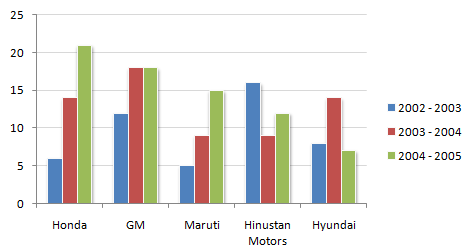# Data Interpretation - Bar Charts - Discussion

The following chart shows the production of cars in thousands.

Production of Cars for 2002 - 2005 period from the Selected Manufacturers1.

How many companies have shown production below their average production in 2002 - 2003, but have showed above the average production in 2003 - 2004 ?

 [A]. One [B]. Two [C]. Three [D]. Four

Explanation:

Average sales of company:

Honda = (6 + 14 + 21)/3 = 13.66

GM = (12 + 18 + 18)/3 = 16

Maruti = (5 + 9 + 15)/3 = 9.66

Hindustan Motors= (16 + 9 + 12)/3 = 12.33

Hyundai = (8 + 14 + 7)/3 = 9.66

 Deepak said: (Oct 8, 2014) Can any body explain this question more clearly?

 Ramakanth said: (Nov 25, 2014) First find out the average of every company for the three years then compare that average with the company's 2002-03 and 2003-04 values.

 Shikha said: (Jun 13, 2015) Please explain this question in detail, According to question I calculated the avg yearly wise. In 2002-03 = Avg production is 11.2. In 2003-4 = Avg production is 9.4. Then How to do find out. "How many companies have shown production below their average production in 2002-2003, but have showed above the average production in 2003-2004".

 Akash said: (Sep 21, 2016) In 2002-03 = Avg is 9.4 In 2003-04 = Avg is 12.6 Then honda Maruti and Hyundai shows below avg and honda gm and Hyundai show above avg. So, the answer is 3 companies.

 Choqi said: (Jul 22, 2018) Honda(2003-04)=14 is above Avg = 13.66. GM(2003-04)=18 is above Avg = 16. Hyundai(2003-04)=14 is above Avg = 9.66. So, Honda + GM + Hyundai = 3.

 Nix said: (Feb 15, 2020) The question is to find which companies show values below their average value in 2002-2003 and above their average value in 2003-2004. First, find average values of all companies and check with 2002-2003 and 2003-2004. Honda= (6+14+21) /3=13.66, in 2002-2003 value is 6 which is below of average value, but in 2003-2004 value is high.

 Deepu said: (Mar 15, 2020) The answer is C. Lets see the explanation: First, find the average production in 2002-3 is 9.4, then find average production in 2003-4 is 12.8 Companies Below Above Honda 6 14 GM No value 12,18 Martuhi 5,9 No value HM 9 16 Hyundai 8 14 In question, they asked below average production in 2002 - 2003 (9.4) and above average production in 2003 - 2004(12.8). From the table, we have to select the company which is having both below and above values. They are Honda, HM, Hyundai.

 Priya said: (Oct 23, 2020) Very helpful, Thank you @Deepu.

 Kiran said: (Aug 31, 2021) Given is average of each company. To get average of entire company: (13.66 + 16 + 9.66 + 12.33 + 9.66)/5 = 12.26.